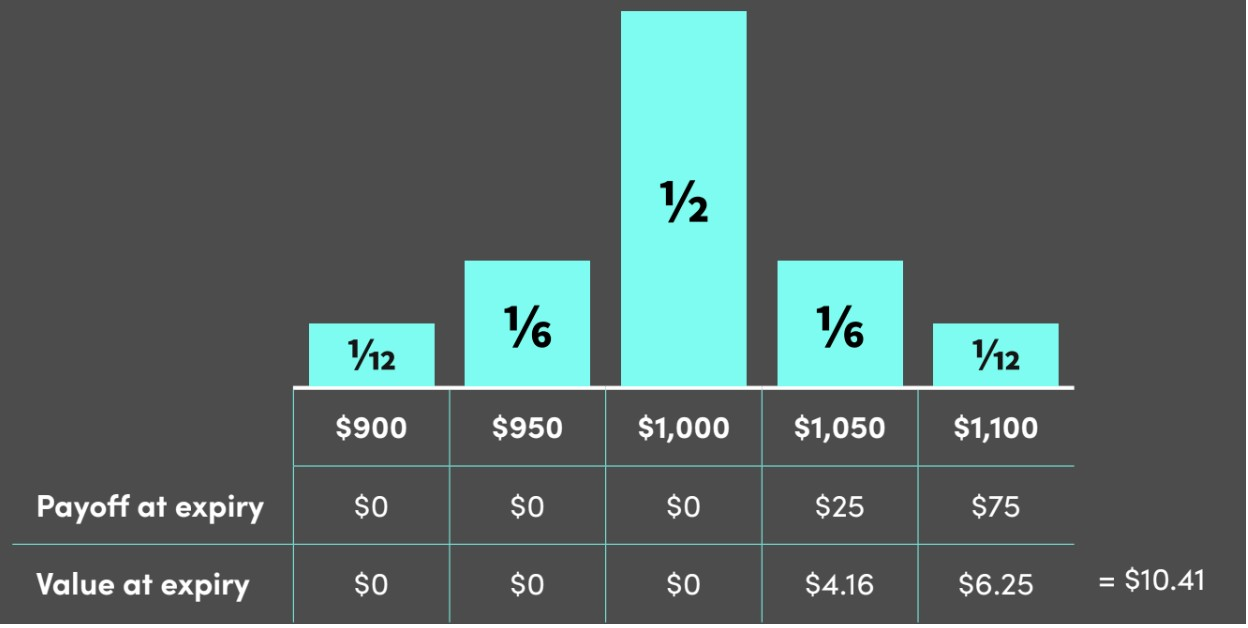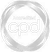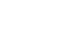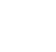# Options Valuation Exercises### Lindsey Matthews

30 years: Risk management & derivatives trading

In this video, Lindsey explains the application of the simple valuation model to value 4 options

Financial MarketsIn this video, Lindsey explains the application of the simple valuation model to value 4 options

Speak to an expert

Speak to an expert today to access this and all of the content on our platform.### Options Valuation Exercises

3 mins 53 secs

Overview

To establish the model distribution you require the forward price and the volatility. The forward price is \$1,000 and the 3-month volatility is 5%.

What is the value of the \$1,000 call?

What is the value of the \$1,000 put?

What is the value of the \$1,025 call?

What is the value of the \$1,025 put?

Key learning objectives:

• Apply the simple valuation model to value 4 options

## Speak to an expert

Speak to an expert today to access this and all of the content on our platform.

Summary

#### What is the value of the \$1,000 call?

First, calculate the payoff at expiry. Only the \$1,050 and \$1,100 bars are in the money, with \$50 and \$100 payoffs respectively.

Second, calculate the value at expiry by multiplying by its probability, which results in a value of \$8.33 for both bars in the money, resulting in an overall value of \$16.66.#### What is the value of the \$1,000 put?

First, calculate the payoff at expiry. Only the \$900 and \$950 bars are in the money, with \$100 and \$50 payoffs respectively.

Second, calculate the value at expiry by multiplying by its probability, which results in a value of \$8.33 for both bars in the money, resulting in an overall value of \$16.33.#### What is the value of the \$1,025 call?

First, calculate the payoff at expiry. Both the \$1,050 and \$1,100 bars are in the money and have a \$25 and \$75 payoff respectively.

Second, calculate the value at expiry by multiplying by its probability, which results in a value of \$4.16 for the \$1,050 bar and \$6.25 for the \$1,100 bar, resulting in an overall value of \$10.41.#### What is the value of the \$1,025 put?

First, calculate the payoff expiry. Both the \$900, \$950, and the \$1,000 bars are in the money, with \$125, \$75 and \$25 payoffs respectively.

Second, calculate the value at expiry by multiplying by its probability, which results in a value of \$10.41, \$12.50 and \$12.50 at each point respectively, resulting in an overall value of \$35.41.

## Speak to an expert

Speak to an expert today to access this and all of the content on our platform.### Lindsey Matthews

Lindsey runs Perfordiant, an investment risk and performance consulting firm. He has worked in financial markets since 1992. Lindsey became an MD in fixed income and equities, ran a Risk function, and was on the management team of an Asset Management fintech business. Lindsey is now a Visiting Fellow at the Henley Business School, and resides on the board of CFA UK.

There are no available videos from "Lindsey Matthews"

### Accredited by